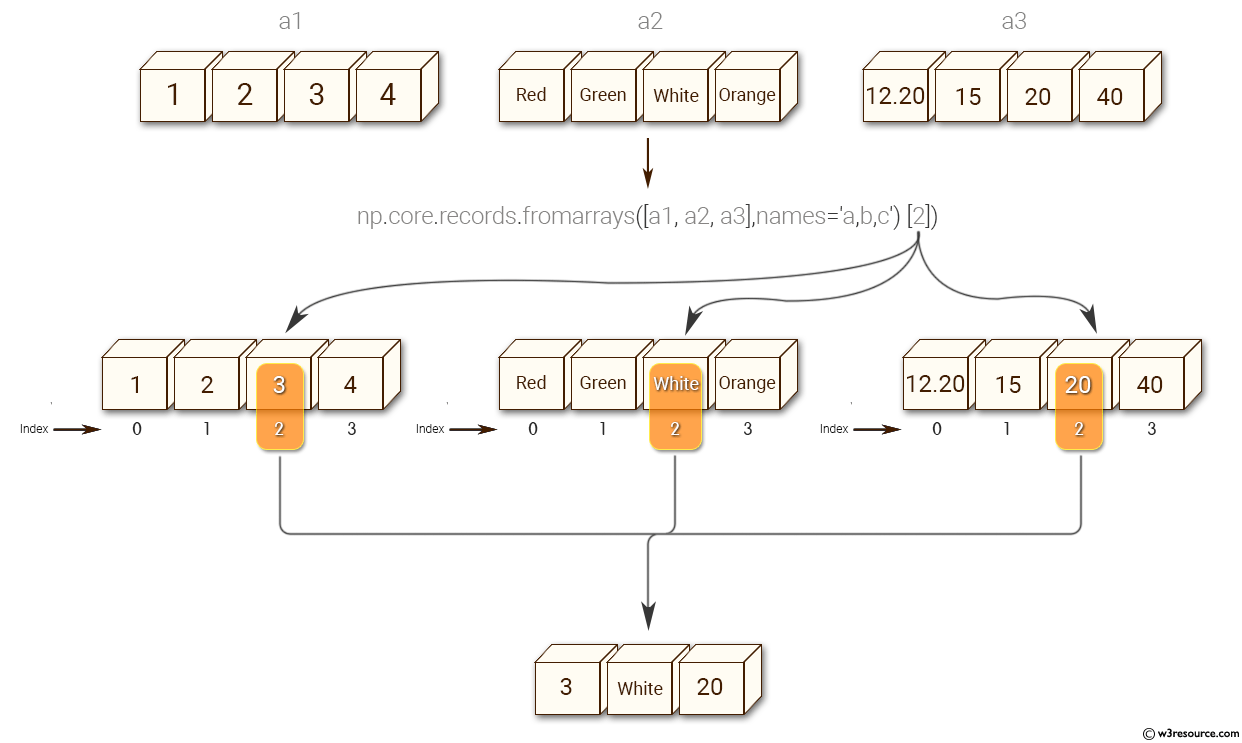﻿ NumPy: Create a record array from a (flat) list of arrays - w3resource# NumPy: Create a record array from a (flat) list of arrays

## NumPy: Array Object Exercise-78 with Solution

Write a NumPy program to create a record array from a (flat) list of arrays.
Sample arrays: [1,2,3,4], ['Red', 'Green', 'White', 'Orange'], [12.20,15,20,40]

Pictorial Presentation:Sample Solution:-

Python Code:

``````import numpy as np
a1=np.array([1,2,3,4])
a2=np.array(['Red','Green','White','Orange'])
a3=np.array([12.20,15,20,40])
result= np.core.records.fromarrays([a1, a2, a3],names='a,b,c')
print(result)
print(result)
print(result)
```
```

Sample Output:

```(1, 'Red', 12.2)
(2, 'Green', 15.0)
(3, 'White', 20.0)
```

Python Code Editor:

Have another way to solve this solution? Contribute your code (and comments) through Disqus.

What is the difficulty level of this exercise?

Test your Python skills with w3resource's quiz

﻿

## Python: Tips of the Day

List comprehension:

```>>> m = [x ** 2 for x in range(5)]
>>> m
[0, 1, 4, 9, 16]
```